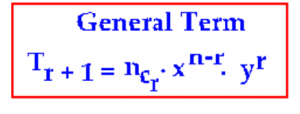General Term in Binomial Expansion (x + y)n isIn order to find any term required in the binomial expansion, we use the General Term.

In (x + y)n, consider the following terms to understand the relation between r and a term T.

The third term is T3 = T2+1 = nc2.xn-2.y2
The fourth term is T4 = T3+1 = nc3. xn–3.y3
The fifth term is T5 = T4+1 = nc4. xn–4 . y4

And so on with the other terms.

Now, observe the following results from the above three terms:

1. (r+1) is the subscript beside T in each of the terms above.
The value of r is 2 in T3, 3 in T4 and 4 in T5 i.e., one less than the term. Thus, to find any term we will need the general term which is Tr+1 , and the value of r is always one less than the general term. For fifth term r is 4, for fourth term r is 3, for third term r is 2 and so on.
2. Therefore Tr+1 is the called the General Term in the binomial expansion. General Term in Binomial Theorem means any term that may be required to be found. When any term in any binomial expansion is to be found, the General Term must be used.
3. Powers of x and y in the general term:• The index (power) of x in the general term is equal to the difference between the superscript n and the subscript r.
• The index (power) of y in the general term is always r .

Combining the above results, we understand and state once again that general term in binomial theorem(which actually means any term in a binomial expansion), is denoted as Tr+1